# 使用C#产生各种随机数（一）

Unity大约 3 分钟

## # 平均分布

### # 演示

``````using System;

namespace RandomDemo
{
public class U
{
Random rand = new Random();

public int U0(int min ,int max)
{
var a = rand.Next(min ,max);
return(a);
}
}
}
``````

``````using System;

namespace RandomDemo
{
class Program
{
static void Main(string[] args)
{
U u = new U();

for (int i = 0; i < 9000; i++)
{
var a = u.U0(-50, 50);
Console.WriteLine(a);
}

}
}
}
``````## # 正态分布

### # 原理### # 演示

``````using System;

namespace RandomDemo
{
public class N
{
Random rand = new Random();

//标准正态分布
public double Normal()
{
double s = 0, u = 0, v = 0;
while (s > 1 || s == 0)
{
u = rand.NextDouble() * 2 - 1;
v = rand.NextDouble() * 2 - 1;

s = u * u + v * v;
}

var z = Math.Sqrt(-2 * Math.Log(s) / s) * u;
return (z);
}

//符合要求的正态分布随机数
public double RandomNormal(double miu ,double sigma)
{
var z = Normal() * sigma + miu;
return (z);
}
}
}
``````

``````using System;

namespace RandomDemo
{
class Program
{
static void Main(string[] args)
{
N n = new N();

for (int i = 0; i < 9000; i++)
{
var z = n.RandomNormal(0, 10);
Console.WriteLine(z);
}

}
}
}
``````## # 下期预告

•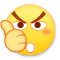0
•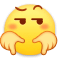0
•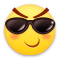0
•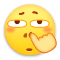0
•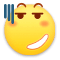0
•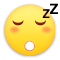0

• 按正序
• 按倒序
• 按热度